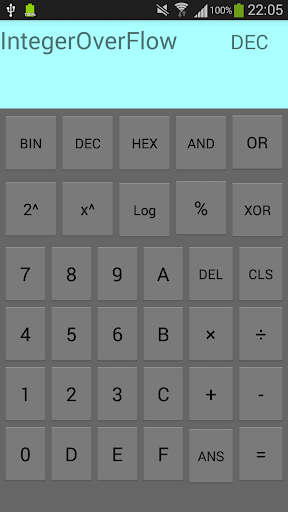# Computer Eng. Calculator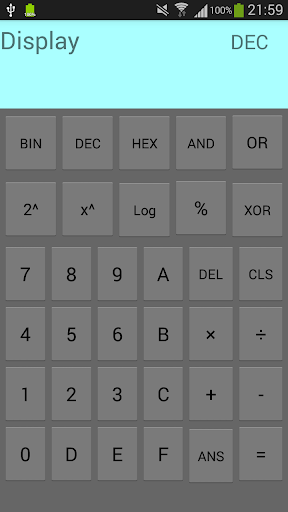A calculator that supports some basics computer engineers operations, which are:

Subtract: -

2 to pow: 2^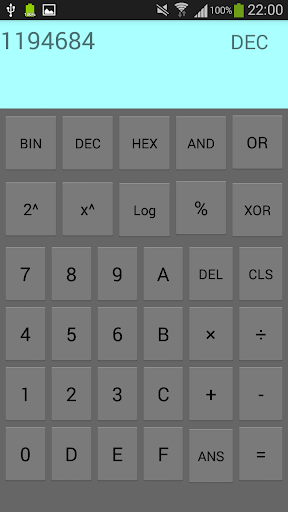x to pow: x^

Logarithm base 2: log

Modulus: %

Logical and: AND "&"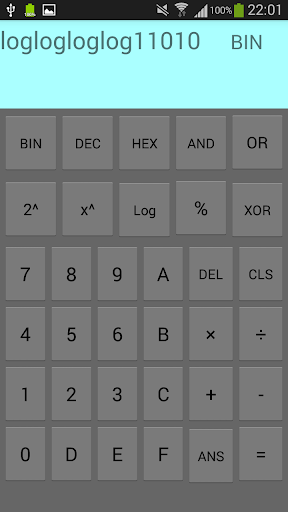Logical or: OR "|"

Logical xor: XOR "⊕"

And also it supports 3 of a the very known bases (instantaneous transformation), which are:

Binary: BIN

Decimal: DEC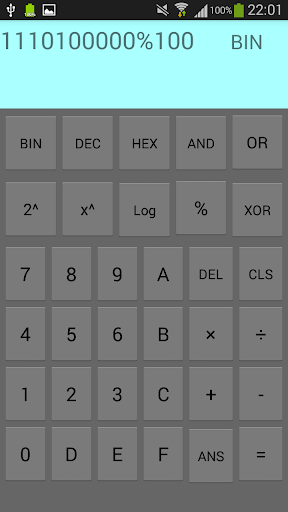A keypad with 1,2,3,4,5,6,7,8,9,0,A,B,C,D,E, and F is provided to enter data.

To evaluate an expression, an equal "=" button is there to done the task.

To delete (backspace) a typo use "DEL" button.

To clear the screen from the whole input use "CLS" button.

To use the last answer found use "ANS" button.

NOTE: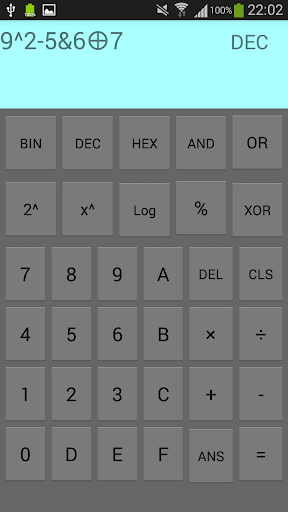1- All logarithm operations are rounded up.

(i.e. loglog .. logloglogn = ceil(log(log( .. log(log(log(n))) .. )))

2- Logarithm function only takes 1 input.

(e.g. in decimal: log64/3 = log(64)/3 = 2)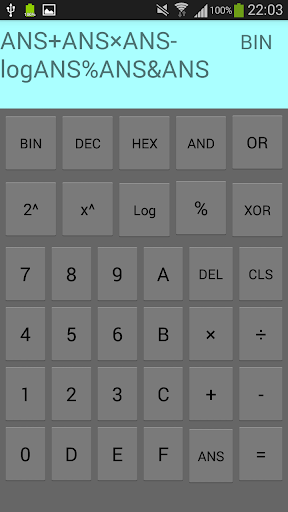3- Arithmetical and Mathematical operations has higher priority than Logical operations.

(e.g. in binary: 1111 - 0011&0001 = 1100&0001 = 1101)

4- The maximum positive number for the result is 2,147,483,646, and if it is exceeded you will get an IntegerOverFlow error, and the minimum negative number of the result is -2,147,483,647, and if it is exceeded you will get an IntegerUnderFlow error.

5- If an input expression is written wrong, a Syntax Error will occurs.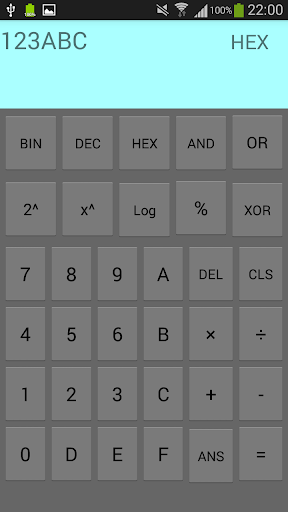(e.g. log+2, &/15).

For developer:

If you are using Java and you need to evaluate some mathematical expressions use "exp4j" library, it is a very simple tool.

Special thanks for "exp4j" creator(s).